#### Inference Principles for Survey Data

Design-based and model-assisted statistical inference for survey data requires that the randomness due to the selection mechanism be taken into account. This can require special estimation principles and techniques.

The SURVEYMEANS, SURVEYFREQ, SURVEYREG, SURVEYLOGISTIC, and SURVEYPHREG procedures support design-based and/or model-assisted inference for sample surveys. Suppose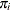is the selection probability for unit i in sample S. The inverse of the inclusion probability is known as sampling weight and is denoted by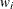. Briefly, the idea is to apply a relationship that exists in the population to the sample and to take into account the sampling weights. For example, to estimate the finite population total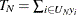based on the sample S, you can accumulate the sampled values while properly weighting: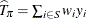. It is easy to verify that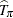is design-unbiased in the sense that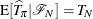(see Cochran 1977).

When a statistical model is present, similar ideas apply. For example, if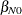and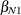are finite population quantities for a simple linear regression working model that minimize the sum of squares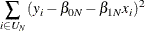in the population, then the sample-based estimators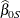and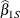are obtained by minimizing the weighted sum of squares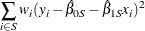in the sample, taking into account the inclusion probabilities.

In model-assisted inference, weighted least squares or pseudo-maximum likelihood estimators are commonly used to solve such estimation problems. Maximum pseudo-likelihood or weighted maximum likelihood estimators for survey data maximize a sample-based estimator of the population likelihood. Assume a working model with uncorrelated responses such that the finite population log-likelihood is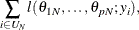where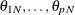are finite population quantities. For independent sampling, one possible sample-based estimator of the population log likelihood is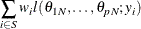Sample-based estimators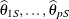are obtained by maximizing this expression.

Design-based and model-based statistical analysis might employ the same statistical model (for example, a linear regression) and the same estimation principle (for example, weighted least squares), and arrive at the same estimates. The design-based estimation of the precision of the estimators differs from the model-based estimation, however. For complex surveys, design-based variance estimates are in general different from their model-based counterpart. The SAS/STAT procedures for survey data (SURVEYMEANS, SURVEYFREQ, SURVEYREG, SURVEYLOGISTIC, and SURVEYPHREG procedures) compute design-based variance estimates for complex survey data. See the section Variance Estimation, in Chapter 14: Introduction to Survey Sampling and Analysis Procedures, for details about design-based variance estimation.